The automatic differentiation guide includes everything required to calculate gradients. This guide focuses on deeper, less common features of the `tf.GradientTape` api.

## Setup

``````import tensorflow as tf

import matplotlib as mpl
import matplotlib.pyplot as plt

mpl.rcParams['figure.figsize'] = (8, 6)
``````

In the automatic differentiation guide you saw how to control which variables and tensors are watched by the tape while building the gradient calculation.

The tape also has methods to manipulate the recording.

If you wish to stop recording gradients, you can use `GradientTape.stop_recording()` to temporarily suspend recording.

This may be useful to reduce overhead if you do not wish to differentiate a complicated operation in the middle of your model. This could include calculating a metric or an intermediate result:

``````x = tf.Variable(2.0)
y = tf.Variable(3.0)

x_sq = x * x
with t.stop_recording():
y_sq = y * y
z = x_sq + y_sq

print('dz/dx:', grad['x'])  # 2*x => 4
``````
```dz/dx: tf.Tensor(4.0, shape=(), dtype=float32)
dz/dy: None

```

If you wish to start over entirely, use `reset()`. Simply exiting the gradient tape block and restarting is usually easier to read, but you can use `reset` when exiting the tape block is difficult or impossible.

``````x = tf.Variable(2.0)
y = tf.Variable(3.0)
reset = True

y_sq = y * y
if reset:
# Throw out all the tape recorded so far
t.reset()
z = x * x + y_sq

print('dz/dx:', grad['x'])  # 2*x => 4
``````
```dz/dx: tf.Tensor(4.0, shape=(), dtype=float32)
dz/dy: None

```

In contrast to the global tape controls above, the `tf.stop_gradient` function is much more precise. It can be used to stop gradients from flowing along a particular path, without needing access to the tape itself:

``````x = tf.Variable(2.0)
y = tf.Variable(3.0)

y_sq = y**2

print('dz/dx:', grad['x'])  # 2*x => 4
``````
```dz/dx: tf.Tensor(4.0, shape=(), dtype=float32)
dz/dy: None

```

In some cases, you may want to control exactly how gradients are calculated rather than using the default. These situations include:

• There is no defined gradient for a new op you are writing.
• The default calculations are numerically unstable.
• You wish to cache an expensive computation from the forward pass.
• You want to modify a value (for example using: `tf.clip_by_value`, `tf.math.round`) without modifying the gradient.

For writing a new op, you can use `tf.RegisterGradient` to set up your own. See that page for details. (Note that the gradient registry is global, so change it with caution.)

For the latter three cases, you can use `tf.custom_gradient`.

Here is an example that applies `tf.clip_by_norm` to the intermediate gradient.

``````# Establish an identity operation, but clip during the gradient pass
def backward(dy):
return tf.clip_by_norm(dy, 0.5)
return y, backward

v = tf.Variable(2.0)
print(t.gradient(output, v))  # calls "backward", which clips 4 to 2

``````
```tf.Tensor(2.0, shape=(), dtype=float32)

```

See the `tf.custom_gradient` decorator for more details.

## Multiple tapes

Multiple tapes interact seamlessly. For example, here each tape watches a different set of tensors:

``````x0 = tf.constant(0.0)
x1 = tf.constant(0.0)

tape0.watch(x0)
tape1.watch(x1)

y0 = tf.math.sin(x0)
y1 = tf.nn.sigmoid(x1)

y = y0 + y1

ys = tf.reduce_sum(y)
``````
``````tape0.gradient(ys, x0).numpy()   # cos(x) => 1.0
``````
```1.0
```
``````tape1.gradient(ys, x1).numpy()   # sigmoid(x1)*(1-sigmoid(x1)) => 0.25
``````
```0.25
```

Operations inside of the `GradientTape` context manager are recorded for automatic differentiation. If gradients are computed in that context, then the gradient computation is recorded as well. As a result, the exact same API works for higher-order gradients as well. For example:

``````x = tf.Variable(1.0)  # Create a Tensorflow variable initialized to 1.0

y = x * x * x

# Compute the gradient inside the outer `t2` context manager
# which means the gradient computation is differentiable as well.

print('dy_dx:', dy_dx.numpy())  # 3 * x**2 => 3.0
print('d2y_dx2:', d2y_dx2.numpy())  # 6 * x => 6.0
``````
```dy_dx: 3.0
d2y_dx2: 6.0

```

While that does give you the second derivative of a scalar function, this pattern does not generalize to produce a Hessian matrix, since `GradientTape.gradient` only computes the gradient of a scalar. To construct a Hessian, see the Hessian example under the Jacobian section.

"Nested calls to `GradientTape.gradient`" is a good pattern when you are calculating a scalar from a gradient, and then the resulting scalar acts as a source for a second gradient calculation, as in the following example.

Many models are susceptible to "adversarial examples". This collection of techniques modifies the model's input to confuse the model's output. The simplest implementation takes a single step along the gradient of the output with respect to the input; the "input gradient".

One technique to increase robustness to adversarial examples is input gradient regularization, which attempts to minimize the magnitude of the input gradient. If the input gradient is small, then the change in the output should be small too.

Below is a naive implementation of input gradient regularization. The implementation is:

1. Calculate the gradient of the output with respect to the input using an inner tape.
2. Calculate the magnitude of that input gradient.
3. Calculate the gradient of that magnitude with respect to the model.
``````x = tf.random.normal([7, 5])

layer = tf.keras.layers.Dense(10, activation=tf.nn.relu)
``````
``````
# The inner tape only takes the gradient with respect to the input,
# not the variables.
t1.watch(x)
y = layer(x)
out = tf.reduce_sum(layer(x)**2)
# 1. Calculate the input gradient.
# 2. Calculate the magnitude of the input gradient.
g1_mag = tf.norm(g1)

# 3. Calculate the gradient of the magnitude with respect to the model.
``````
``````[var.shape for var in dg1_mag]
``````
```[TensorShape([5, 10]), TensorShape()]
```

## Jacobians

All the previous examples took the gradients of a scalar target with respect to some source tensor(s).

The Jacobian matrix represents the gradients of a vector valued function. Each row contains the gradient of one of the vector's elements.

The `GradientTape.jacobian` method allows you to efficiently calculate a Jacobian matrix.

Note that:

• Like `gradient`: The `sources` argument can be a tensor or a container of tensors.
• Unlike `gradient`: The `target` tensor must be a single tensor.

### Scalar source

As a first example, here is the Jacobian of a vector-target with respect to a scalar-source.

``````x = tf.linspace(-10.0, 10.0, 200+1)
delta = tf.Variable(0.0)

y = tf.nn.sigmoid(x+delta)

dy_dx = tape.jacobian(y, delta)
``````

When you take the Jacobian with respect to a scalar the result has the shape of the target, and gives the gradient of the each element with respect to the source:

``````print(y.shape)
print(dy_dx.shape)
``````
```(201,)
(201,)

```
``````plt.plot(x.numpy(), y, label='y')
plt.plot(x.numpy(), dy_dx, label='dy/dx')
plt.legend()
_ = plt.xlabel('x')
``````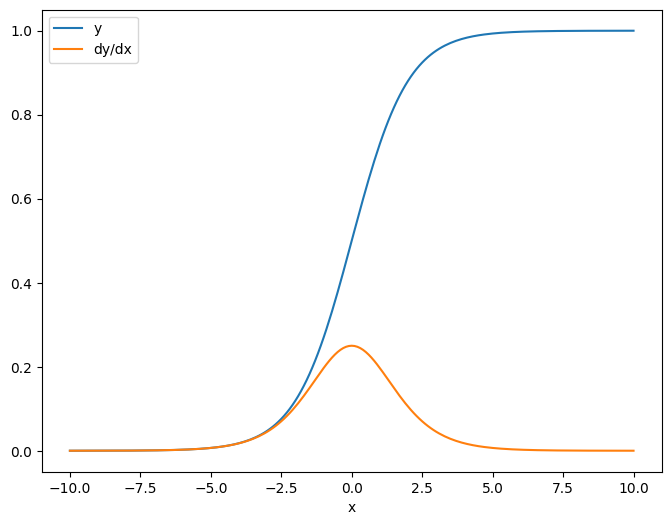### Tensor source

Whether the input is scalar or tensor, `GradientTape.jacobian` efficiently calculates the gradient of each element of the source with respect to each element of the target(s).

For example, the output of this layer has a shape of `(10, 7)`:

``````x = tf.random.normal([7, 5])
layer = tf.keras.layers.Dense(10, activation=tf.nn.relu)

y = layer(x)

y.shape
``````
```TensorShape([7, 10])
```

And the layer's kernel's shape is `(5, 10)`:

``````layer.kernel.shape
``````
```TensorShape([5, 10])
```

The shape of the Jacobian of the output with respect to the kernel is those two shapes concatenated together:

``````j = tape.jacobian(y, layer.kernel)
j.shape
``````
```TensorShape([7, 10, 5, 10])
```

If you sum over the target's dimensions, you're left with the gradient of the sum that would have been calculated by `GradientTape.gradient`:

``````g = tape.gradient(y, layer.kernel)
print('g.shape:', g.shape)

j_sum = tf.reduce_sum(j, axis=[0, 1])
delta = tf.reduce_max(abs(g - j_sum)).numpy()
assert delta < 1e-3
print('delta:', delta)
``````
```g.shape: (5, 10)
delta: 2.3841858e-07

```

#### Example: Hessian

While `tf.GradientTape` doesn't give an explicit method for constructing a Hessian matrix it's possible to build one using the `GradientTape.jacobian` method.

``````x = tf.random.normal([7, 5])
layer1 = tf.keras.layers.Dense(8, activation=tf.nn.relu)
layer2 = tf.keras.layers.Dense(6, activation=tf.nn.relu)

x = layer1(x)
x = layer2(x)
loss = tf.reduce_mean(x**2)

h = t2.jacobian(g, layer1.kernel)
``````
``````print(f'layer.kernel.shape: {layer1.kernel.shape}')
print(f'h.shape: {h.shape}')
``````
```layer.kernel.shape: (5, 8)
h.shape: (5, 8, 5, 8)

```

To use this Hessian for a Newton's method step, you would first flatten out its axes into a matrix, and flatten out the gradient into a vector:

``````n_params = tf.reduce_prod(layer1.kernel.shape)

g_vec = tf.reshape(g, [n_params, 1])
h_mat = tf.reshape(h, [n_params, n_params])
``````

The Hessian matrix should be symmetric:

``````def imshow_zero_center(image, **kwargs):
lim = tf.reduce_max(abs(image))
plt.imshow(image, vmin=-lim, vmax=lim, cmap='seismic', **kwargs)
plt.colorbar()
``````
``````imshow_zero_center(h_mat)
``````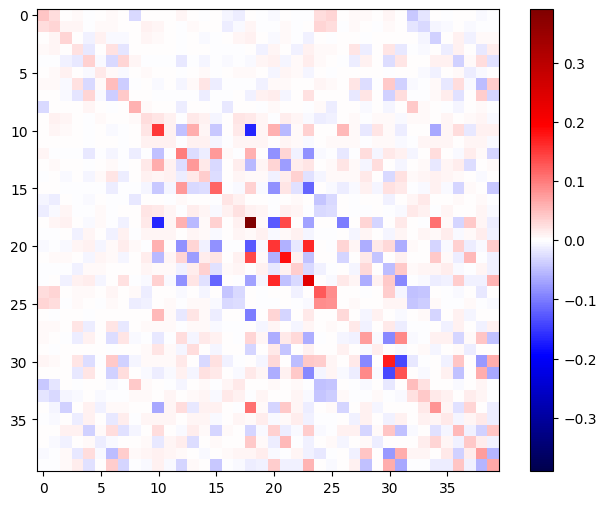The Newton's method update step is shown below.

``````eps = 1e-3
eye_eps = tf.eye(h_mat.shape)*eps
``````
``````# X(k+1) = X(k) - (∇²f(X(k)))^-1 @ ∇f(X(k))
# h_mat = ∇²f(X(k))
# g_vec = ∇f(X(k))
update = tf.linalg.solve(h_mat + eye_eps, g_vec)

# Reshape the update and apply it to the variable.
_ = layer1.kernel.assign_sub(tf.reshape(update, layer1.kernel.shape))
``````

While this is relatively simple for a single `tf.Variable`, applying this to a non-trivial model would require careful concatenation and slicing to produce a full Hessian across multiple variables.

### Batch Jacobian

In some cases, you want to take the Jacobian of each of a stack of targets with respect to a stack of sources, where the Jacobians for each target-source pair are independent.

For example, here the input `x` is shaped `(batch, ins)` and the output `y` is shaped `(batch, outs)`.

``````x = tf.random.normal([7, 5])

layer1 = tf.keras.layers.Dense(8, activation=tf.nn.elu)
layer2 = tf.keras.layers.Dense(6, activation=tf.nn.elu)

tape.watch(x)
y = layer1(x)
y = layer2(y)

y.shape
``````
```TensorShape([7, 6])
```

The full Jacobian of `y` with respect to `x` has a shape of `(batch, ins, batch, outs)`, even if you only want `(batch, ins, outs)`.

``````j = tape.jacobian(y, x)
j.shape
``````
```TensorShape([7, 6, 7, 5])
```

If the gradients of each item in the stack are independent, then every `(batch, batch)` slice of this tensor is a diagonal matrix:

``````imshow_zero_center(j[:, 0, :, 0])
_ = plt.title('A (batch, batch) slice')
``````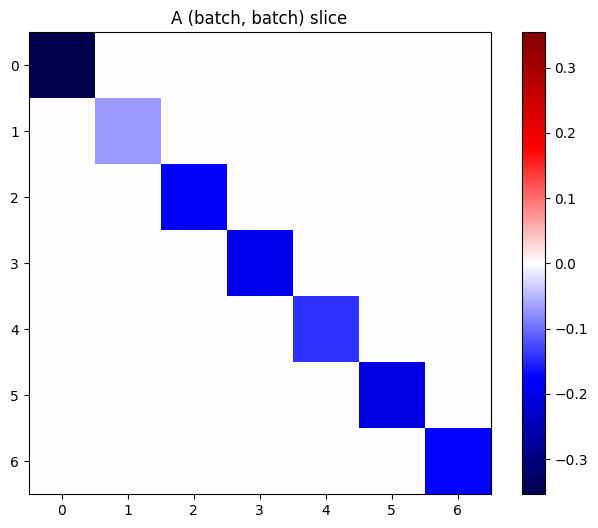``````def plot_as_patches(j):
# Reorder axes so the diagonals will each form a contiguous patch.
j = tf.transpose(j, [1, 0, 3, 2])
# Pad in between each patch.
lim = tf.reduce_max(abs(j))
j = tf.pad(j, [[0, 0], [1, 1], [0, 0], [1, 1]],
constant_values=-lim)
# Reshape to form a single image.
s = j.shape
j = tf.reshape(j, [s*s, s*s])
imshow_zero_center(j, extent=[-0.5, s-0.5, s-0.5, -0.5])

plot_as_patches(j)
_ = plt.title('All (batch, batch) slices are diagonal')
``````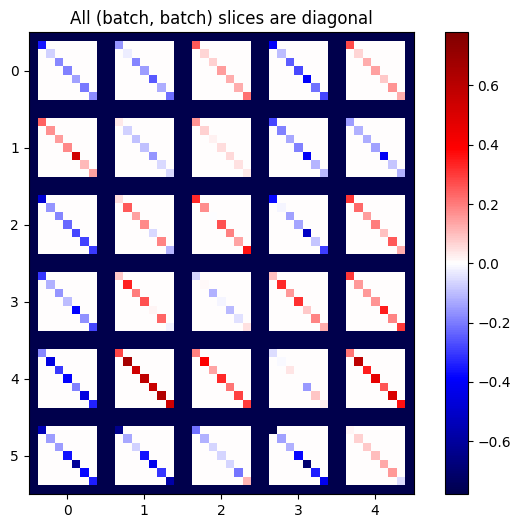To get the desired result you can sum over the duplicate `batch` dimension, or else select the diagonals using `tf.einsum`.

``````j_sum = tf.reduce_sum(j, axis=2)
print(j_sum.shape)
j_select = tf.einsum('bxby->bxy', j)
print(j_select.shape)
``````
```(7, 6, 5)
(7, 6, 5)

```

It would be much more efficient to do the calculation without the extra dimension in the first place. The `GradientTape.batch_jacobian` method does exactly that.

``````jb = tape.batch_jacobian(y, x)
jb.shape
``````
```TensorShape([7, 6, 5])
```
``````error = tf.reduce_max(abs(jb - j_sum))
assert error < 1e-3
print(error.numpy())
``````
```0.0

```
``````x = tf.random.normal([7, 5])

layer1 = tf.keras.layers.Dense(8, activation=tf.nn.elu)
bn = tf.keras.layers.BatchNormalization()
layer2 = tf.keras.layers.Dense(6, activation=tf.nn.elu)

tape.watch(x)
y = layer1(x)
y = bn(y, training=True)
y = layer2(y)

j = tape.jacobian(y, x)
print(f'j.shape: {j.shape}')
``````
```j.shape: (7, 6, 7, 5)

```
``````plot_as_patches(j)

_ = plt.title('These slices are not diagonal')
_ = plt.xlabel("Don't use `batch_jacobian`")
``````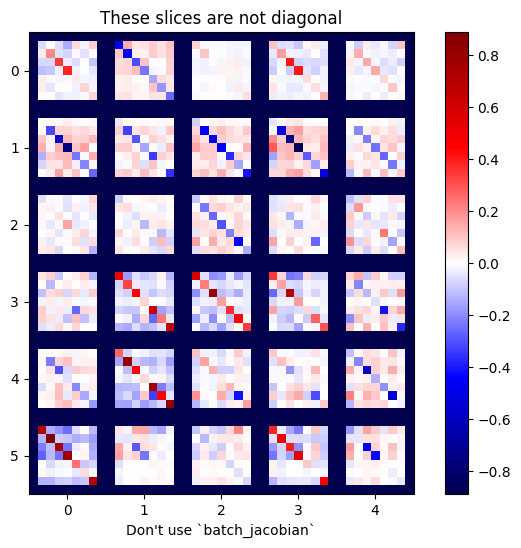In this case `batch_jacobian` still runs and returns something with the expected shape, but it's contents have an unclear meaning.

``````jb = tape.batch_jacobian(y, x)
print(f'jb.shape: {jb.shape}')
``````
```jb.shape: (7, 6, 5)

```